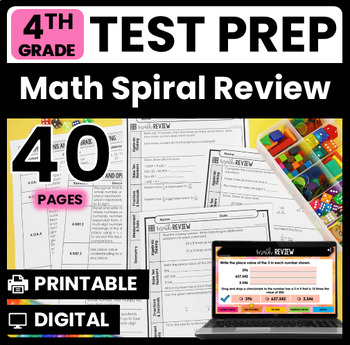# 4th Grade Math Test Prep Review | Google Slides™ Math for Distance Learning4th
Subjects
Standards
Resource Type
Formats Included
• PDF
•Google Apps™
PagesThe Teacher-Author indicated this resource includes assets from Google Workspace (e.g. docs, slides, etc.).

### Description

Do you need a 4th grade math test prep review that covers all of common core math skills? This test prep math resource includes 40 pages of 4th grade math review specifically written for the common core math standards.

NOTE: This resource now has a Google Slides version, making it ideal for digital distance learning and remote teaching! Please see the 3rd page of the PDF for details and the link!

This math review resource was created to serve as a spiral review of 4th grade common core math standards prior to state testing.

✏ 40 pages of review of all 4th grade common core standards/domains

✏ Skill alignment

⚠️This resource was not created as a spiral review to use throughout the entire year as it would be impossible to match it to pacing.

I personally use this 4th grade math test prep review the 40 days leading up to our state assessment. Using it in this way helps me not only review the standards, but also target skills for re-teaching. I also use this to target specific students who need re-teaching. I use this math test prep resource for small group re-teaching.

✏ The math test prep review pages are organized by domain. Each section of the review pages contains problems for a different domain of the CCSS standards. There is a checklist at the end of each set that shows which standards are reviewed on which page.

Using the 4th Grade Math Test Prep

The review pages can be used for

• morning work
• homework
• daily review
• test prep mini-lesson
• math centers
• independent practice

The digital version contains exactly the same content as the printable version, but has been redesigned to work digitally in Google Slides. Digital access can be found on pages 3-4 of the PDF. For a sneak peek of how the digital version works, be sure to watch the video preview.

More 4th Grade Math Test Prep Resources

Math Test Prep MEGA Bundle

Math No Prep Test Prep Helpers

Math Test Prep Centers

Math Printable Review Booklet

Jennifer Findley

You may also like:

Standards Based Math Center Bundle (Includes over 80 Centers!)

Differentiated Math Skill Sheets for Every Standard (Differentiated at 3 Levels)

Roll and Answer Low Prep Math Centers

Math Choice Boards

Math Sorts

Total Pages
Included
Teaching Duration
2 months
Report this Resource to TpT
Reported resources will be reviewed by our team. Report this resource to let us know if this resource violates TpT’s content guidelines.

### Standards

to see state-specific standards (only available in the US).
Know relative sizes of measurement units within one system of units including km, m, cm; kg, g; lb, oz.; l, ml; hr, min, sec. Within a single system of measurement, express measurements in a larger unit in terms of a smaller unit. Record measurement equivalents in a two-column table. For example, know that 1 ft is 12 times as long as 1 in. Express the length of a 4 ft snake as 48 in. Generate a conversion table for feet and inches listing the number pairs (1, 12), (2, 24), (3, 36),...
Use the four operations to solve word problems involving distances, intervals of time, liquid volumes, masses of objects, and money, including problems involving simple fractions or decimals, and problems that require expressing measurements given in a larger unit in terms of a smaller unit. Represent measurement quantities using diagrams such as number line diagrams that feature a measurement scale.
Apply the area and perimeter formulas for rectangles in real world and mathematical problems. For example, find the width of a rectangular room given the area of the flooring and the length, by viewing the area formula as a multiplication equation with an unknown factor.
Make a line plot to display a data set of measurements in fractions of a unit (1/2, 1/4, 1/8). Solve problems involving addition and subtraction of fractions by using information presented in line plots. For example, from a line plot find and interpret the difference in length between the longest and shortest specimens in an insect collection.
Recognize angles as geometric shapes that are formed wherever two rays share a common endpoint, and understand concepts of angle measurement: